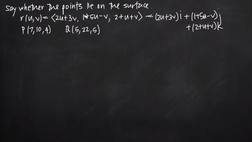PLAY PREVIEW

### Segments in this Video

#### Points That Lie on a Particular Surface(01:05)

FREE PREVIEW

To find whether or not a given set of points lies on a particular surface, you need to find the parametric equations of the surface.

#### Constructing a Parametric Equation(01:12)

To determine whether or not a point lies on the surface represented by a parametric equation, plug in the coordinates into the equation to determine if it evaluates to a valid value.

#### Solving Simultaneous Equations(03:03)

Simultaneous equations are two or more algebraic equations that share variables and can be solved at the same time. There can be infinite possible solutions.

#### Concluding the Equation(02:00)

To check your answer, plug in both values into either of the original equations.

#### Credits(00:00)

Credits

For additional digital leasing and purchase options contact a media consultant at 800-257-5126
(press option 3) or sales@films.com.

# Points on the surface

Part of the Series : Integral Calc: Calculus 3
 3-Year Streaming Price: \$49.95

Share

### Description

This video tutorial works through math problems/equations that address topics in Calculus 3, Vectors. This specific tutorial addresses Points on the surface.

Length: 8 minutes

Item#: BVL275745Closed Captioned